Proportional Relationships Stoichiometry mass relationships between substances in

• Slides: 11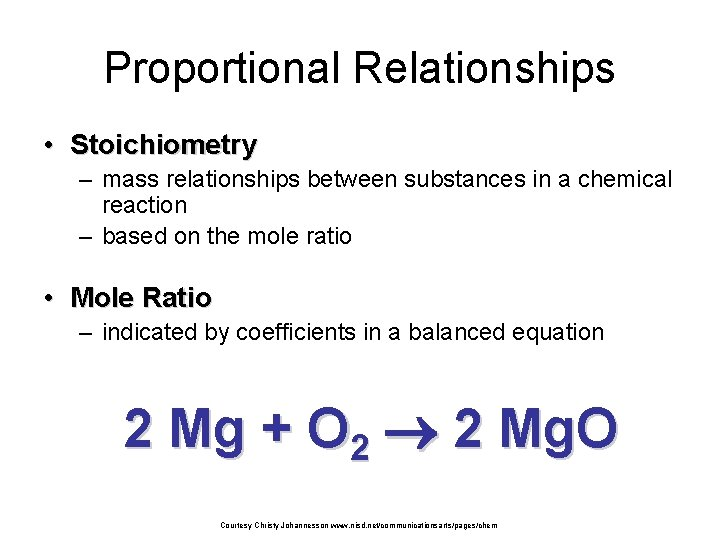Proportional Relationships • Stoichiometry – mass relationships between substances in a chemical reaction – based on the mole ratio • Mole Ratio – indicated by coefficients in a balanced equation 2 Mg + O 2 2 Mg. O Courtesy Christy Johannesson www. nisd. net/communicationsarts/pages/chem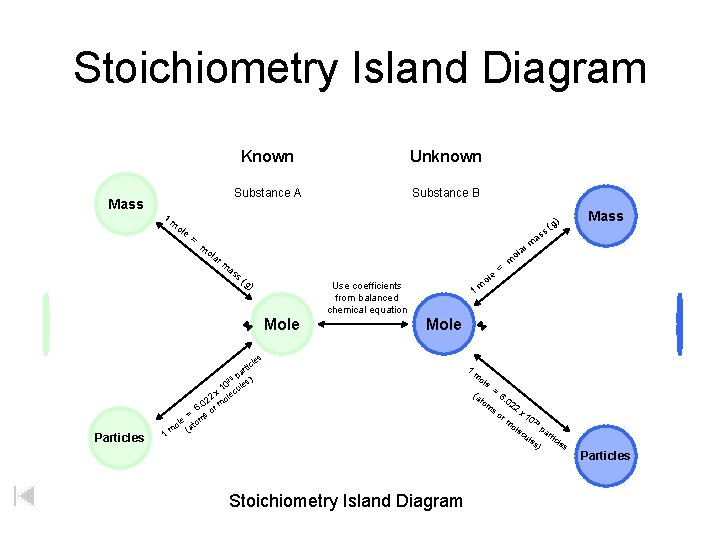Stoichiometry Island Diagram Mass 1 Known Unknown Substance A Substance B ole = m as a ol s( g) Use coefficients from balanced chemical equation Mole e ol m = m 1 Mole les 1 Mass a rm m ola r Particles ) (g ss m c rti pa ) 23 0 les x 1 lecu 2 o 02 6. or m = s m ole (ato m Stoichiometry Island Diagram 1 m ole (a to m = 6. 02 so 2 x 1 0 23 ole pa cu r les ticle s ) rm Particles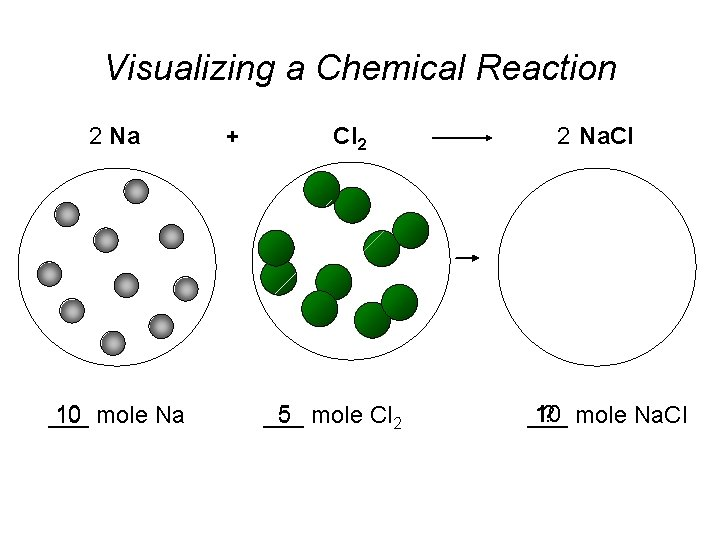Visualizing a Chemical Reaction 2 Na 10 mole Na ___ + Cl 2 5 mole Cl 2 ___ 2 Na. Cl 10 ? mole Na. Cl ___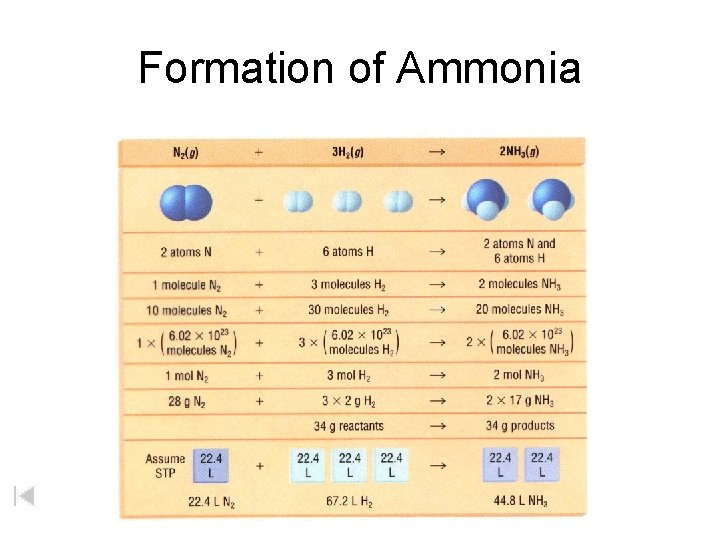Formation of AmmoniaStoichiometry Steps 1. Write a balanced equation. 2. Identify known & unknown. 3. Line up conversion factors. – Mole ratio moles Molarratio mass moles grams – Mole - moles – Avogadro’s number - particles moles Core step in all stoichiometry problems!! 4. Check answer. Courtesy Christy Johannesson www. nisd. net/communicationsarts/pages/chemStoichiometry Problems • How many moles of KCl. O 3 must decompose in order to produce 9 moles of oxygen gas? 2 KCl. O 3 2 KCl + 3 O 2 ? mol 9 mol O 2 2 mol KCl. O 3 3 mol O 2 9 mol = 6 mol KCl. O 3 Courtesy Christy Johannesson www. nisd. net/communicationsarts/pages/chem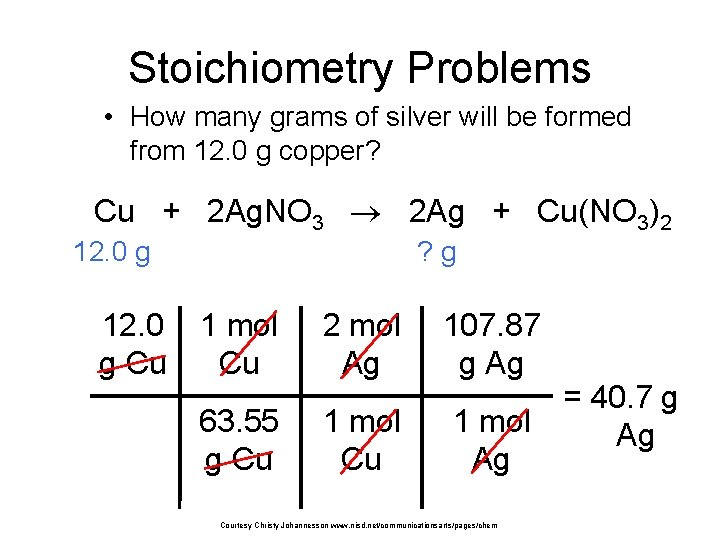Stoichiometry Problems • How many grams of silver will be formed from 12. 0 g copper? Cu + 2 Ag. NO 3 2 Ag + Cu(NO 3)2 12. 0 g Cu ? g 1 mol Cu 2 mol Ag 107. 87 g Ag 63. 55 g Cu 1 mol Ag Courtesy Christy Johannesson www. nisd. net/communicationsarts/pages/chem = 40. 7 g Ag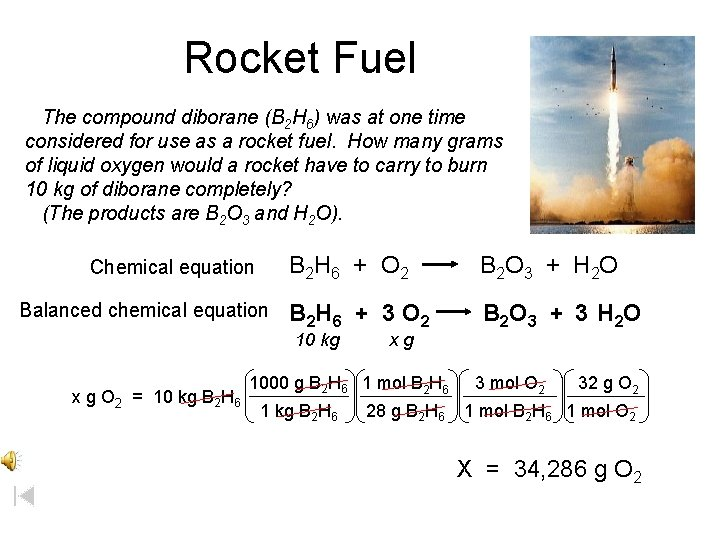Rocket Fuel The compound diborane (B 2 H 6) was at one time considered for use as a rocket fuel. How many grams of liquid oxygen would a rocket have to carry to burn 10 kg of diborane completely? (The products are B 2 O 3 and H 2 O). Chemical equation Balanced chemical equation B 2 H 6 + O 2 B 2 O 3 + H 2 O B 2 H 6 + 3 O 2 B 2 O 3 + 3 H 2 O 10 kg x g O 2 = 10 kg B 2 H 6 xg 1000 g B 2 H 6 1 mol B 2 H 6 1 kg B 2 H 6 28 g B 2 H 6 3 mol O 2 32 g O 2 1 mol B 2 H 6 1 mol O 2 X = 34, 286 g O 2Limiting Reactants • Limiting Reactant – used up in a reaction – determines the amount of product • Excess Reactant – added to ensure that the other reactant is completely used up – cheaper & easier to recycle Courtesy Christy Johannesson www. nisd. net/communicationsarts/pages/chemPercent Yield measured in lab % yield = actual yield theoretical yield calculated on paper x 100When 45. 8 g of K 2 CO 3 react with excess HCl, 46. 3 g of KCl are formed. Calculate theoretical and % yields of KCl. actual yield 46. 3 g K 2 CO 3 + 2 HCl 2 KCl + H 2 O + CO 2 45. 8 g excess ? g theoretical yield Theoretical yield x g KCl = 45. 8 g K 2 CO 3 % Yield = 1 mol K 2 CO 3 2 mol KCl 74. 5 g KCl = 49. 4 g KCl 1 mol K CO 1 mol KCl 138 g K 2 CO 3 2 3 Actual Yield Theoretical Yield % Yield = 46. 3 g KCl x 100 % Yield = 93. 7% efficient